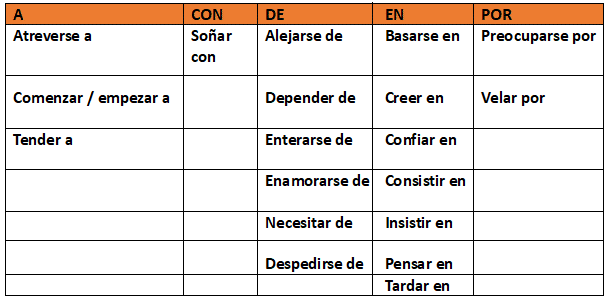Published on: Grammar Bites

# Spanish prepositions and el régimen preposicional

The complemento de régimen preposicional is a sentence element that’s introduced by a preposition when required by the verb.

This complemento conveys basic information necessary to complete the meaning of the verb. In other words, if we take out the complemento out of the sentence, something would be missing; the meaning of the verb would not be complete. That’s why some Spanish verbs require this complemento de régimen preposicional.

###### How can I know when a verb requires un complemento de régimen preposicional?

There’s an easy formula to help us figure this out using the following question: ¿preposición + qué + verbo?

For example:

• Camila sueña con casarse de blanco.
• ¿Con qué sueña Camila?
• Camila sueña con casarse de blanco = Complemento de régimen preposicional

If we take out the complemento “con casarse de blanco”, we would just know that Camila has a dream, but we would not know what it is. We need the complemento to complete the idea.

• Yo pienso en ti a cada momento.
• ¿En qué pienso?
• Pienso en ti = Complemento de régimen preposicional

If we take out the complemento “en ti”, all the other person would know is that we think, plain and simple. They wouldn’t know that we’re thinking about them. We need the complemento to complete the idea.

• Ellos tardaron mucho en llegar.
• ¿En qué tardaron ellos?
• Tardaron en llegar. = Complemento de régimen preposicional

If we take out the complemento “en llegar”, we would know that they took a long time to do something, but we wouldn’t know what that something is. We need the complemento to complete the idea.

• Ellos tienden a comer sopas calientes en el invierno.
• ¿A qué tienden ellos?
• Ellos tienden a comer sopas. = Complemento de régimen preposicional

If we take out the complemento “a comer sopas”, we would know that they tend to to do something, but we wouldn’t know what that something is. We need the complemento to complete the idea.

Let’s take a look at some frequently-used verbs that require el complemento de régimen preposicional:###### Important:

We must be careful because not all elements introduced by prepositions will be complementos de régimen preposicional. Sometimes we’ll have a complemento circunstancial de lugar (introduced by the preposition EN) o de tiempo (ntroduced by the prepositions A, EN), or we can have a direct or indirect object which are introduced by the prepositions A or Para when talking about people.

• Yo invité a mis amigos a la fiesta. = Objeto directo.
• Yo vivo en el campo. = Complemento circunstancial (In this case, la preposición EN indicates location; it’s not part of the verb).
• La fiesta comenzó a las 7 p.m. = Complemento circunstancial de tiempo

## This content is locked